# 电视剧里的代码真能运行吗？

### 剧中代码赏析

1. 首先是路人同学的代码：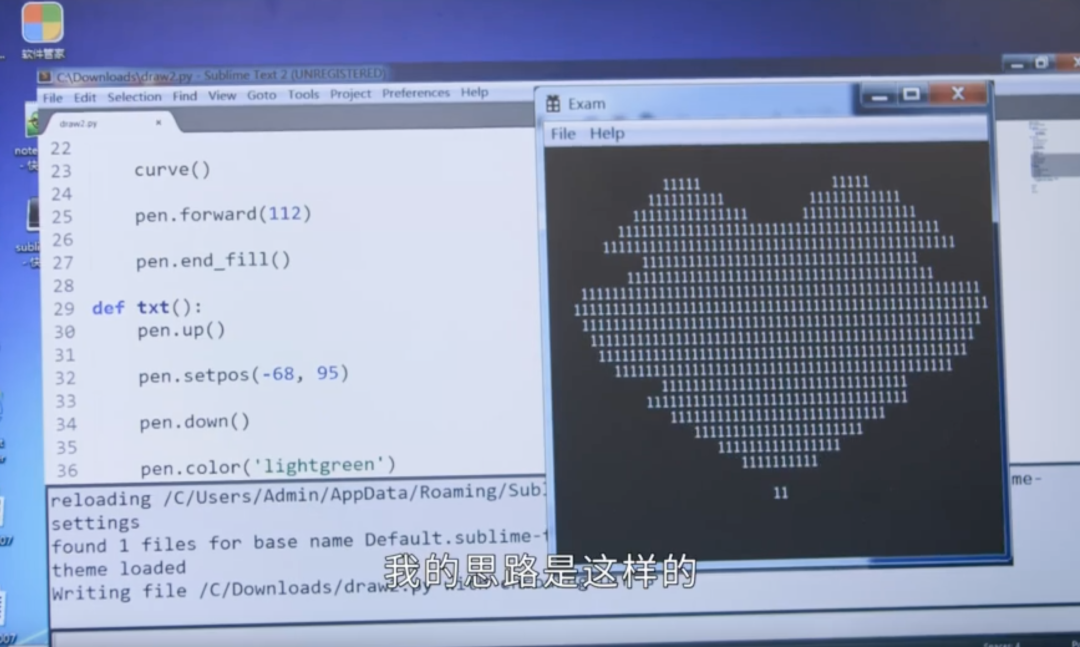``````import turtle as t
t.color('red')
t.begin_fill()
t.circle(-100, 170)
t.circle(-300, 40)
t.right(38)
t.circle(-300, 40)
t.circle(-100, 170)
t.end_fill()
t.done()``````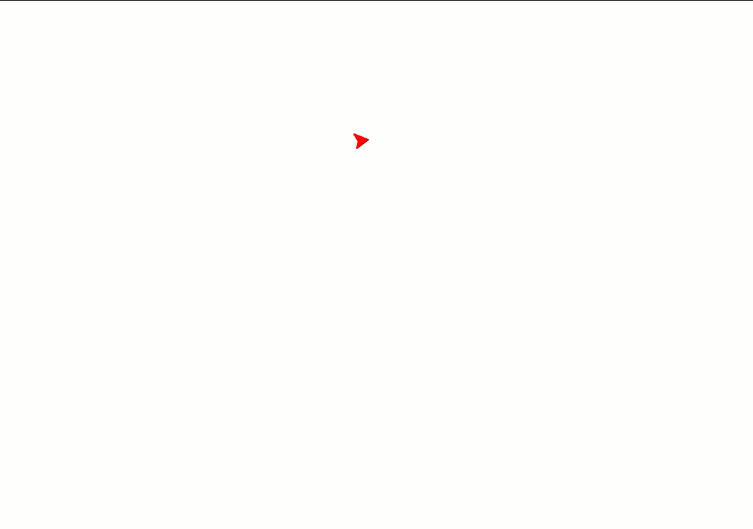2. 然后是课代表向路人同学展示的优秀代码：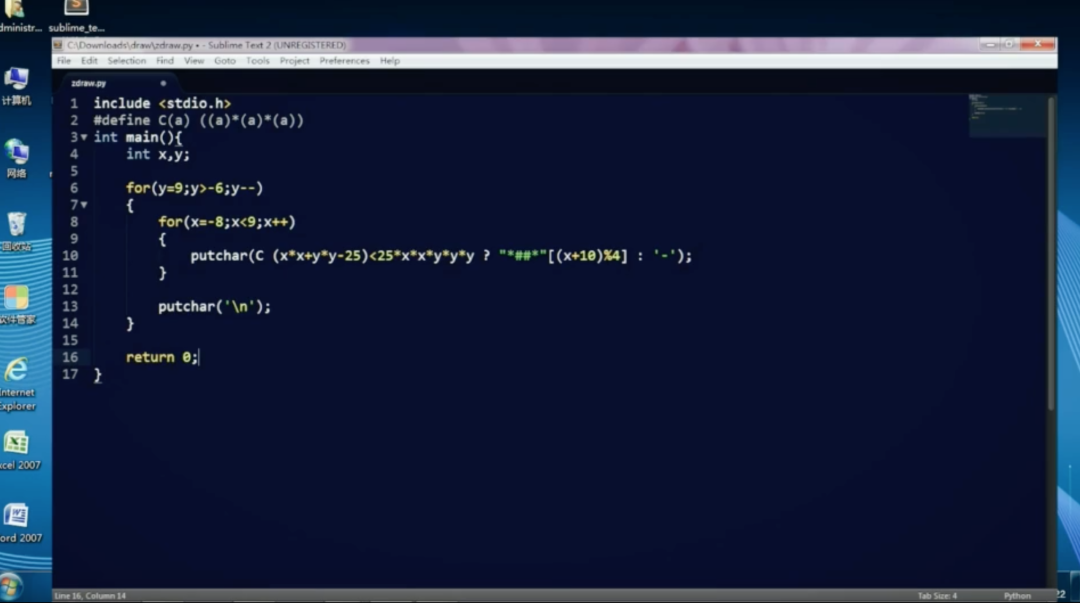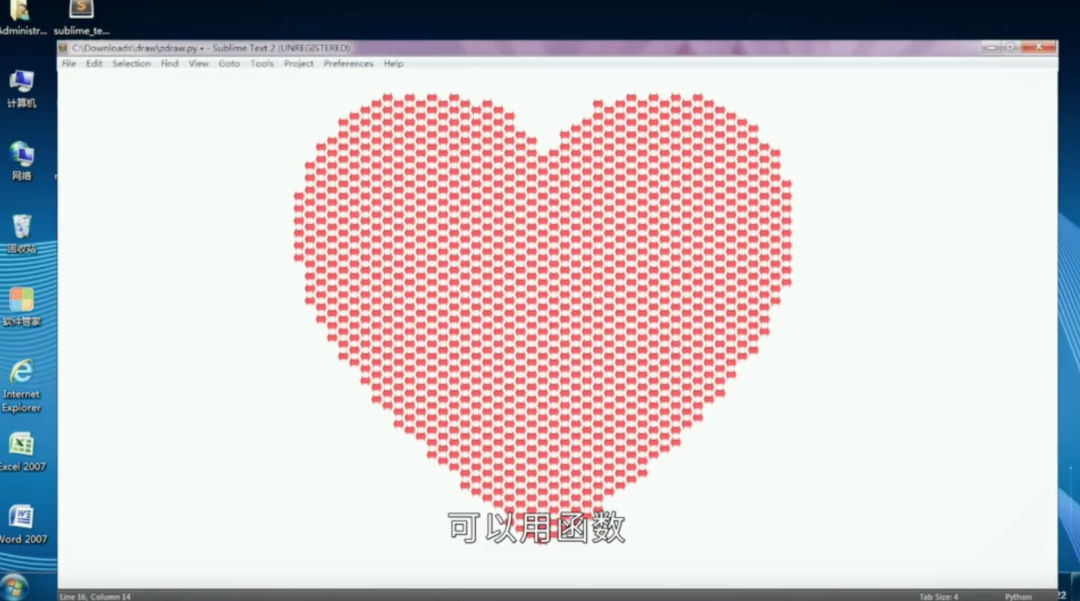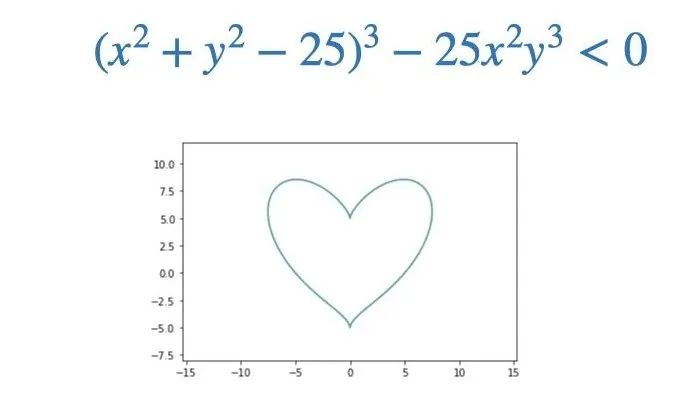``````for y in range(9, -6, -1):
for x in range(-8, 9):
print('*##*'[(x+10)%4] if (x*x+y*y-25)**3 < 25*x*x*y*y*y else '-', end=' ')
print()``````

​​​​​​效果：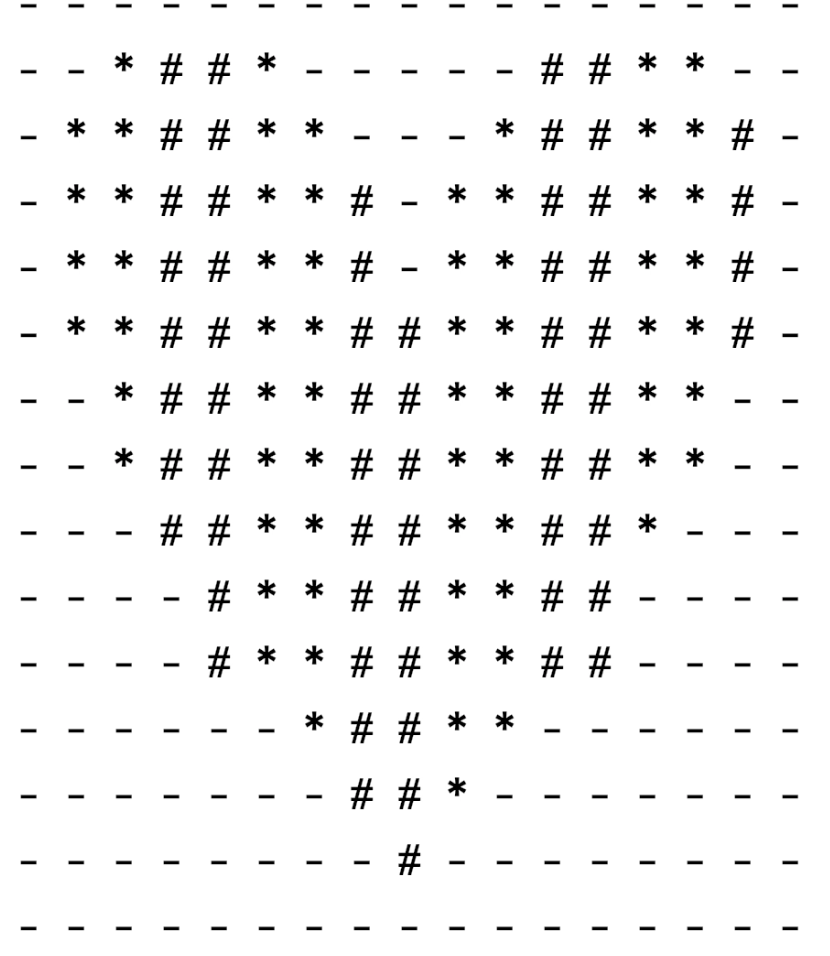``````for y in range(9, -6, -1):
for x in range(-8, 9):
print('1' if (x*x+y*y-25)**3 < 25*x*x*y*y*y else ' ', end=' ')
print()``````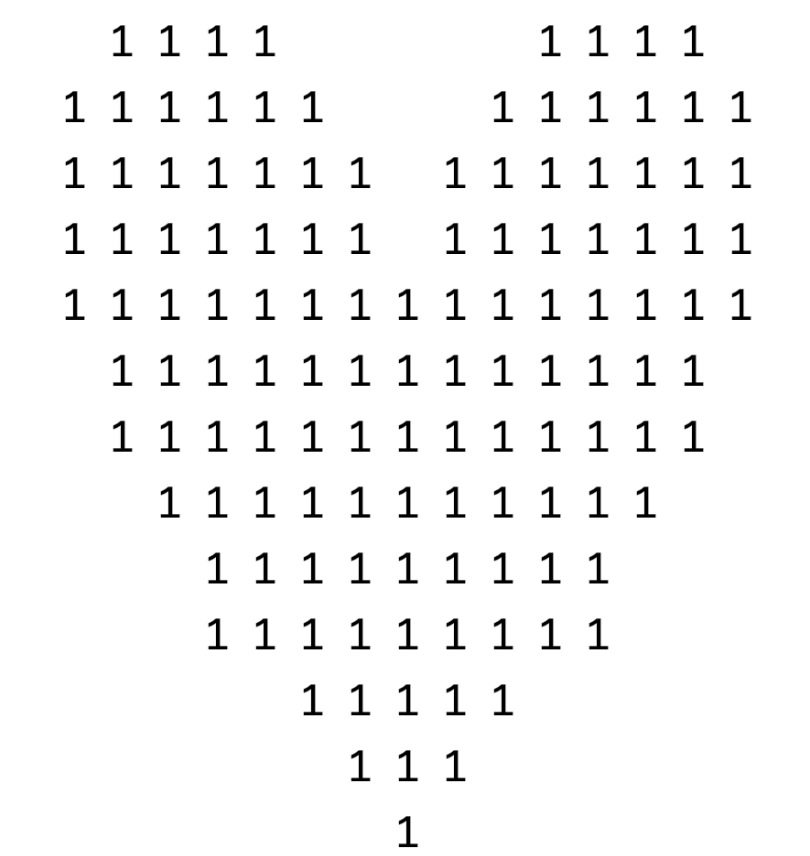3. 最后是主角狂拽酷炫D炸天的跳动爱心：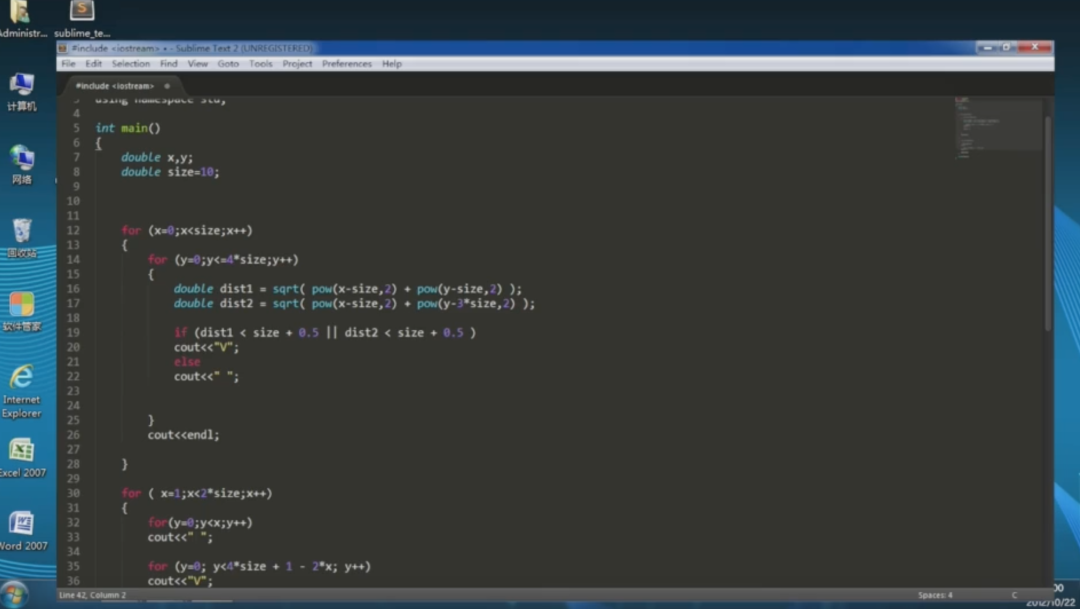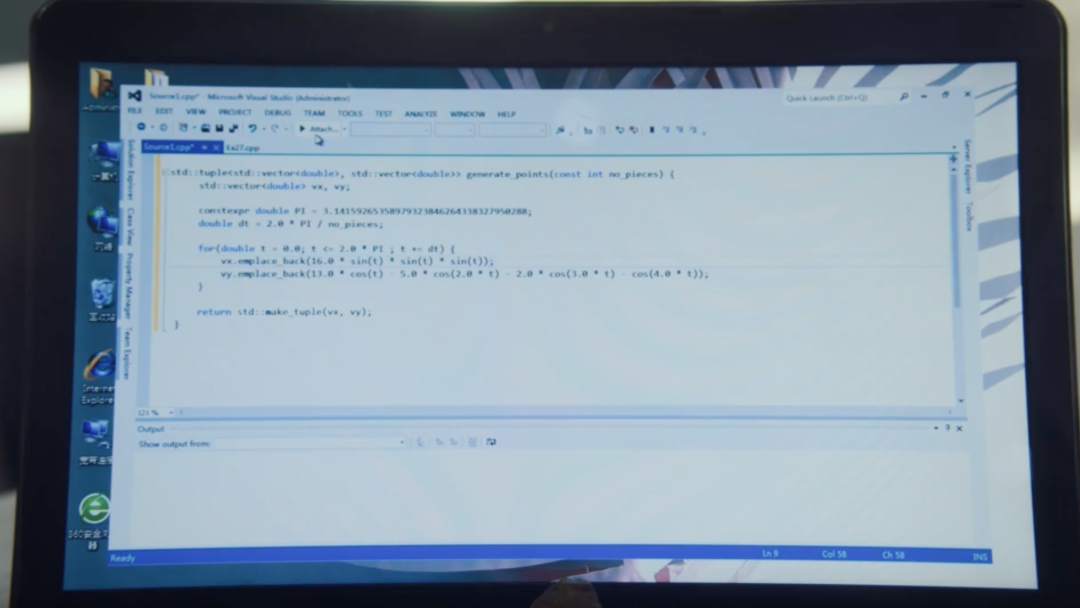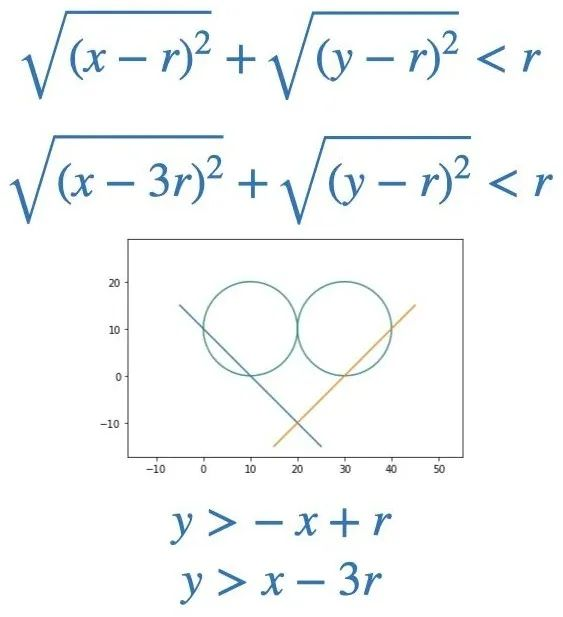``````size = 10
for x in range(size):
for y in range(4*size+1):
dist1 = ((x-size)**2 + (y-size)**2) ** 0.5
dist2 = ((x-size)**2 + (y-3*size)**2) ** 0.5
if dist1 < size + 0.5 or dist2 < size + 0.5:
print('V', end=' ')
else:
print(' ', end=' ')
print()

for x in range(1, 2*size):
for y in range(x):
print(' ', end=' ')
for y in range(4*size+1-2*x):
print('V', end=' ')
print()``````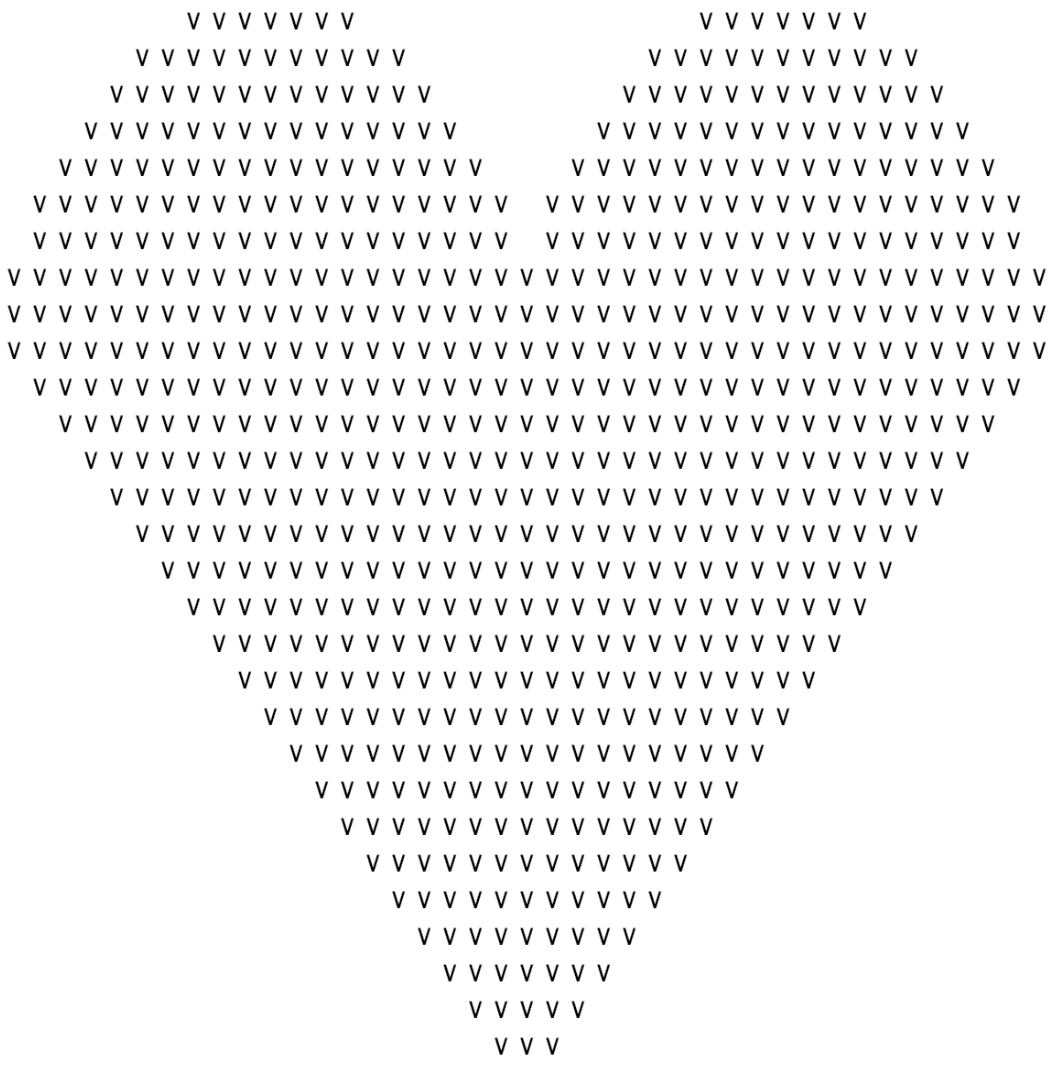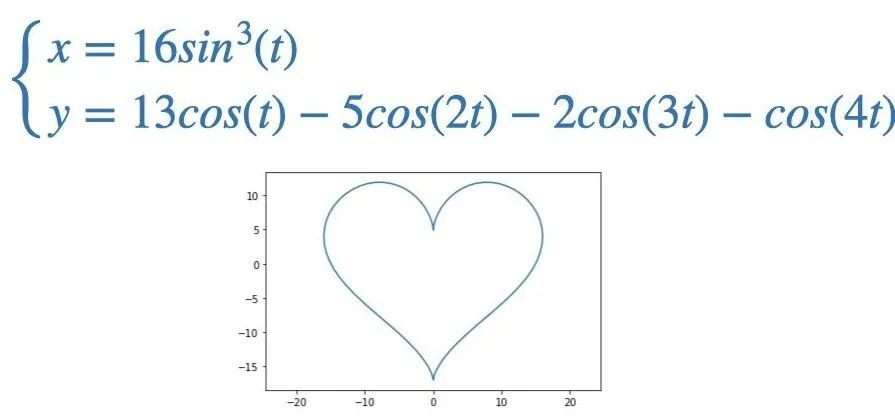``````from math import pi, sin, cos
import matplotlib.pyplot as plt
no_pieces = 100
dt = 2*pi/no_pieces
t = 0
vx = []
vy = []
while t <= 2*pi:
vx.append(16*sin(t)**3)
vy.append(13*cos(t)-5*cos(2*t)-2*cos(3*t)-cos(4*t))
t += dt
plt.plot(vx, vy)
plt.show()``````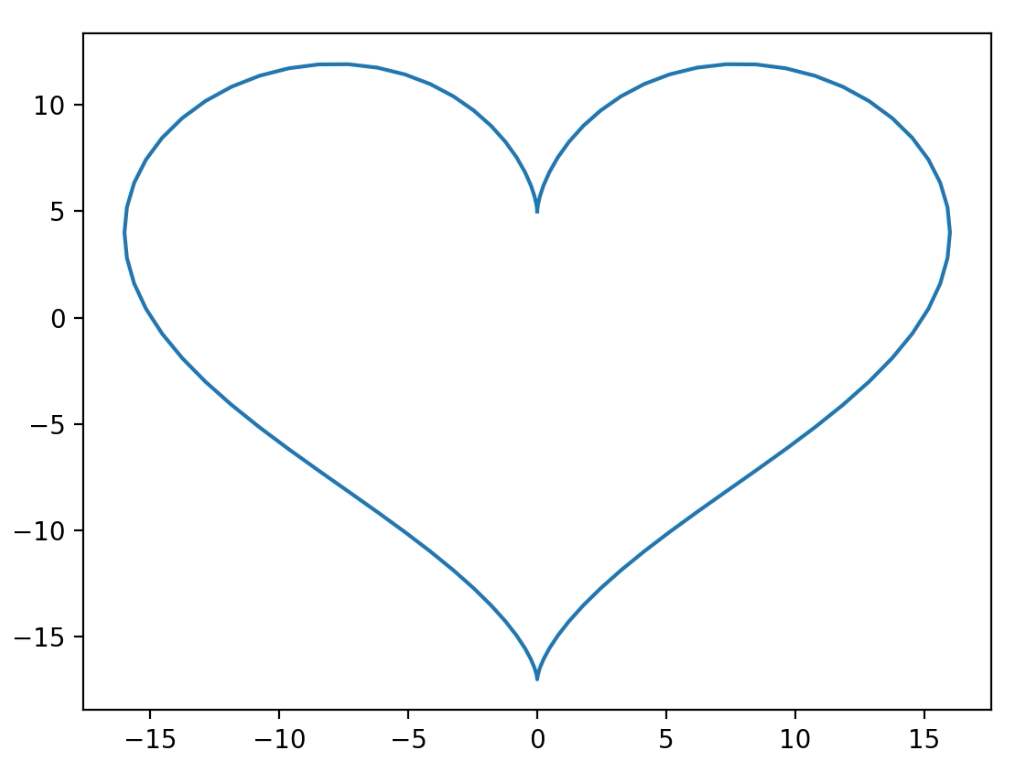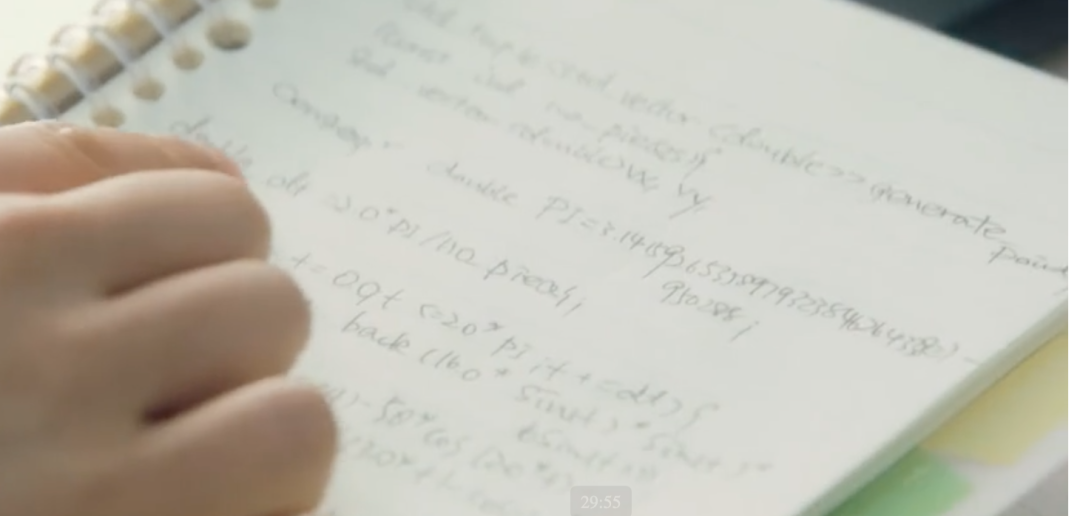### 跳动爱心实现

1. 想要绘制动态的效果，必定要借助一些库的帮助，不然代码量肯定会让你感动得想哭。这里我们将使用之前 羊了个羊游戏 里用过的 pgzero 库。然后结合最后那个极坐标爱心曲线代码，先绘制出曲线上离散的点。

``````import pgzrun
from math import pi, sin, cos

no_p = 100
dt = 2*3/no_p
t = 0
x = []
y = []
while t <= 2*3:
x.append(16*sin(t)**3)
y.append(13*cos(t)-5*cos(2*t)-2*cos(3*t)-cos(4*t))
t += dt

def draw():
screen.clear()
for i in range(len(x)):
screen.draw.filled_rect(Rect((x[i]*10+400, -y[i]*10+300), (4, 4)), 'pink')

pgzrun.go()``````2. 把点的数量增加，同时沿着原点到每个点的径向加一个随机数，并且这个随机数是按照正态分布来的（半个正态分布），大概率分布在曲线上，向曲线内部递减。这样，就得到这样一个随机分布的爱心效果。

``````...
no_p = 20000
...
while t <= 2*pi:
l = 10 - abs(random.gauss(10, 2) - 10)
x.append(l*16*sin(t)**3)
y.append(l*(13*cos(t)-5*cos(2*t)-2*cos(3*t)-cos(4*t)))
t += dt
...``````3. 下面就是让点动起来，这步是关键，也有一点点复杂。为了方便对于每个点进行控制，这里将每个点自定义成了一个Particle类的实例。

``````class Particle():
def __init__(self, pos, size, f):
self.pos = pos
self.pos0 = pos
self.size = size
self.f = f

def draw(self):
screen.draw.filled_rect(Rect((10*self.f*self.pos + 400, -10*self.f*self.pos + 300), self.size), 'hot pink')

def update(self, t):
df = 1 + (2 - 1.5) * sin(t * 3) / 8
self.pos = self.pos0 * df, self.pos0 * df

...

t = 0
def draw():
screen.clear()
for p in particles:
p.draw()

def update(dt):
global t
t += dt
for p in particles:
p.update(t)``````4. 剧中爱心跳动时，靠中间的点波动的幅度更大，有一种扩张的效果。所以再根据每个点距离原点的远近，再加上一个系数，离得越近，系数越大。

``````class Particle():
...
def update(self, t):
df = 1 + (2 - 1.5 * self.f) * sin(t * 3) / 8
self.pos = self.pos0 * df, self.pos0 * df``````5. 最后再用同样的方法画一个更大一点的爱心，这个爱心不需要跳动，只要每一帧随机绘制就可以了。

``````def draw():
...
t = 0
while t < 2*pi:
f = random.gauss(1.1, 0.1)
x = 16*sin(t)**3
y = 13*cos(t)-5*cos(2*t)-2*cos(3*t)-cos(4*t)
size = (random.uniform(0.5,2.5), random.uniform(0.5,2.5))
screen.draw.filled_rect(Rect((10*f*x + 400, -10*f*y + 300), size), 'hot pink')
t += dt * 3``````